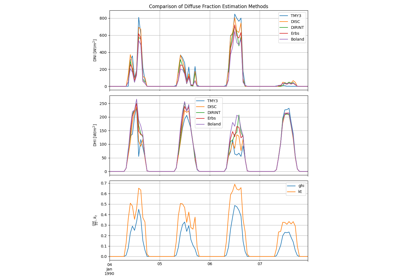Use the component sum equations to calculate the missing series, using the other available time series. One of the three parameters (ghi, dhi, dni) is passed as None, and the other associated series passed are used to calculate the missing series value.

The “component sum” or “closure” equation relates the three primary irradiance components as follows:

$GHI = DHI + DNI \cos(\theta_z)$
Parameters
• solar_zenith (Series) – Zenith angles in decimal degrees, with datetime index. Angles must be >=0 and <=180. Must have the same datetime index as ghi, dhi, and dni series, when available.

• ghi (Series, optional) – Pandas series of dni data, with datetime index. Must have the same datetime index as dni, dhi, and zenith series, when available.

• dhi (Series, optional) – Pandas series of dni data, with datetime index. Must have the same datetime index as ghi, dni, and zenith series, when available.

• dni (Series, optional) – Pandas series of dni data, with datetime index. Must have the same datetime index as ghi, dhi, and zenith series, when available.

• dni_clear (Series, optional) – Pandas series of clearsky dni data. Must have the same datetime index as ghi, dhi, dni, and zenith series, when available. See dni() for details.

Returns

component_sum_df (Dataframe) – Pandas series of ‘ghi’, ‘dhi’, and ‘dni’ columns with datetime index

## Examples using pvlib.irradiance.complete_irradiance#Diffuse Fraction Estimation

Diffuse Fraction Estimation